# JP4297501B2 - Moving object periphery monitoring device - Google Patents

## Info

Publication number
JP4297501B2
JP4297501B2 JP2004234946A JP2004234946A JP4297501B2 JP 4297501 B2 JP4297501 B2 JP 4297501B2 JP 2004234946 A JP2004234946 A JP 2004234946A JP 2004234946 A JP2004234946 A JP 2004234946A JP 4297501 B2 JP4297501 B2 JP 4297501B2
Authority
JP
Japan
Prior art keywords
image
plane
step
object
vpp
Prior art date
Legal status (The legal status is an assumption and is not a legal conclusion. Google has not performed a legal analysis and makes no representation as to the accuracy of the status listed.)
Active
Application number
JP2004234946A
Other languages
Japanese (ja)
Other versions
JP2006054681A (en
Inventor

Original Assignee

Priority date (The priority date is an assumption and is not a legal conclusion. Google has not performed a legal analysis and makes no representation as to the accuracy of the date listed.)
Filing date
Publication date
Application filed by 国立大学法人東京工業大学, 本田技研工業株式会社 filed Critical 国立大学法人東京工業大学
Priority to JP2004234946A priority Critical patent/JP4297501B2/en
Publication of JP2006054681A publication Critical patent/JP2006054681A/en
Application granted granted Critical
Publication of JP4297501B2 publication Critical patent/JP4297501B2/en
Active legal-status Critical Current
Anticipated expiration legal-status Critical

• 238000000034 methods Methods 0 claims description 87
• 238000004364 calculation methods Methods 0 claims description 61
• 230000000875 corresponding Effects 0 claims description 41
• 238000000605 extraction Methods 0 claims description 18
• 238000003384 imaging method Methods 0 claims description 14
• 230000001595 contractor Effects 0 claims description 5
• 239000011159 matrix materials Substances 0 description 136
• 238000006243 chemical reaction Methods 0 description 23
• 238000001914 filtration Methods 0 description 2
• 210000000887 Face Anatomy 0 description 1
• 238000000354 decomposition Methods 0 description 1
• 238000000926 separation method Methods 0 description 1

## Images

•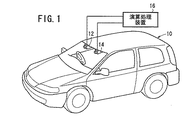•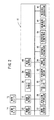•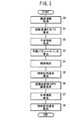•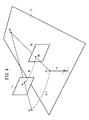•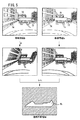•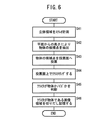•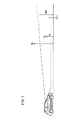•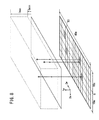•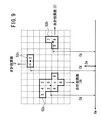•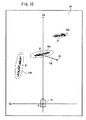•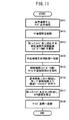•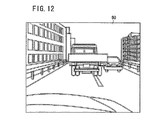•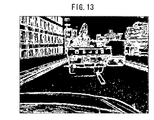•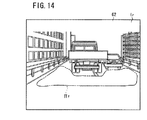•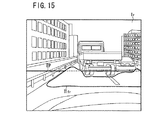•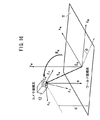•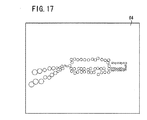•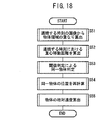•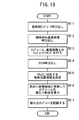•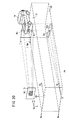••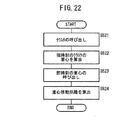•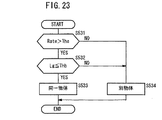•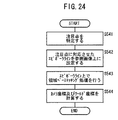•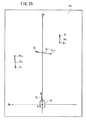•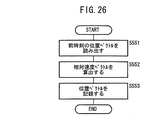•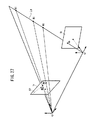•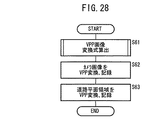•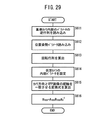•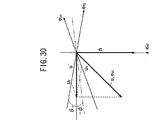•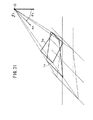•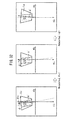•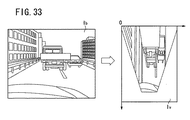•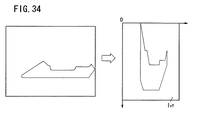•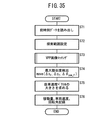••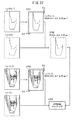•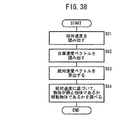•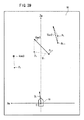## Classifications

• GPHYSICS
• G06COMPUTING; CALCULATING; COUNTING
• G06KRECOGNITION OF DATA; PRESENTATION OF DATA; RECORD CARRIERS; HANDLING RECORD CARRIERS
• G06K9/00Methods or arrangements for reading or recognising printed or written characters or for recognising patterns, e.g. fingerprints
• G06K9/00624Recognising scenes, i.e. recognition of a whole field of perception; recognising scene-specific objects
• G06K9/00791Recognising scenes perceived from the perspective of a land vehicle, e.g. recognising lanes, obstacles or traffic signs on road scenes
• G06K9/00805Detecting potential obstacles
• BPERFORMING OPERATIONS; TRANSPORTING
• B60VEHICLES IN GENERAL
• B60RVEHICLES, VEHICLE FITTINGS, OR VEHICLE PARTS, NOT OTHERWISE PROVIDED FOR
• B60R1/00Optical viewing arrangements
• GPHYSICS
• G06COMPUTING; CALCULATING; COUNTING
• G06TIMAGE DATA PROCESSING OR GENERATION, IN GENERAL
• G06T7/00Image analysis
• G06T7/70Determining position or orientation of objects or cameras
• G06T7/73Determining position or orientation of objects or cameras using feature-based methods
• G06T7/74Determining position or orientation of objects or cameras using feature-based methods involving reference images or patches
• HELECTRICITY
• H04ELECTRIC COMMUNICATION TECHNIQUE
• H04NPICTORIAL COMMUNICATION, e.g. TELEVISION
• H04N7/00Television systems
• H04N7/18Closed circuit television systems, i.e. systems in which the signal is not broadcast
• H04N7/181Closed circuit television systems, i.e. systems in which the signal is not broadcast for receiving images from a plurality of remote sources
• BPERFORMING OPERATIONS; TRANSPORTING
• B60VEHICLES IN GENERAL
• B60RVEHICLES, VEHICLE FITTINGS, OR VEHICLE PARTS, NOT OTHERWISE PROVIDED FOR
• B60R2300/00Details of viewing arrangements using cameras and displays, specially adapted for use in a vehicle
• B60R2300/10Details of viewing arrangements using cameras and displays, specially adapted for use in a vehicle characterised by the type of camera system used
• B60R2300/107Details of viewing arrangements using cameras and displays, specially adapted for use in a vehicle characterised by the type of camera system used using stereoscopic cameras
• BPERFORMING OPERATIONS; TRANSPORTING
• B60VEHICLES IN GENERAL
• B60RVEHICLES, VEHICLE FITTINGS, OR VEHICLE PARTS, NOT OTHERWISE PROVIDED FOR
• B60R2300/00Details of viewing arrangements using cameras and displays, specially adapted for use in a vehicle
• B60R2300/80Details of viewing arrangements using cameras and displays, specially adapted for use in a vehicle characterised by the intended use of the viewing arrangement
• B60R2300/8093Details of viewing arrangements using cameras and displays, specially adapted for use in a vehicle characterised by the intended use of the viewing arrangement for obstacle warning
• GPHYSICS
• G06COMPUTING; CALCULATING; COUNTING
• G06KRECOGNITION OF DATA; PRESENTATION OF DATA; RECORD CARRIERS; HANDLING RECORD CARRIERS
• G06K9/00Methods or arrangements for reading or recognising printed or written characters or for recognising patterns, e.g. fingerprints
• G06K9/20Image acquisition
• G06K9/32Aligning or centering of the image pick-up or image-field
• G06K2009/3291Pattern tracking

## Description

The present invention relates to a moving object periphery monitoring apparatus that stably identifies an area corresponding to a road plane from an acquired image and calculates the position of an object in a short time.

In the guidance of a moving body such as an automobile, a method for detecting a road plane area that can be traveled or detecting an obstacle present in the travel environment includes a method using laser radar, ultrasonic waves, millimeter wave radar, and the like, It can be roughly divided into methods using images.

In the detection method using laser radar or millimeter wave radar, there is a problem that the apparatus is generally expensive and sufficient spatial resolution cannot be obtained. In addition, the detection method using ultrasonic waves has a problem in that it is difficult to measure far and the spatial resolution is low.

On the other hand, the method using an image can be divided into a method using a single eye and a method using a compound eye. Conventionally, most of the methods using an image use a single eye, that is, an image obtained from one viewpoint, and are mainly used in a developed environment such as an expressway, and white lines (for example, separation lines) And the center line) are detected to detect the travel area. However, in general roads and parking lots where the presence of white lines etc. is not guaranteed, and the road surface colors and patterns vary, only the density pattern obtained from the monocular image can be used to stabilize the driveable area and obstacles. It is difficult to distinguish.

On the other hand, in the detection method using compound eyes, that is, a stereo image, since the three-dimensional structure of the environment can be used in principle, there is a possibility that the driving environment can be recognized more stably. In particular, since the travelable area can be regarded as almost a plane in the space, a projective transformation matrix between images is calculated, and one image is two-dimensionally projected and superimposed on the other image using this projective transformation matrix. A method for detecting whether or not the image is a planar region or an obstacle from the overlapping state of images has been proposed (see Patent Document 1 and Non-Patent Document 1).

By the way, in order to detect other objects from a moving body such as a vehicle, it is desirable to perform detection in as short a time as possible and perform real-time processing. However, the conventional stereo matching method based on image processing requires a large amount of computation. Yes Processing speed is slow.

From such a viewpoint, a stereo matching method has been proposed in which an edge portion of an image is extracted from a stereo image, and then a straight edge having a predetermined length or more is selected to recognize an object (for example, see Patent Document 2). .

JP 2000-293893 A JP 2004-30453 A Heung-Yung Shum, Richard Szeliski, "Panoramic Image Mosaics" Technical Report, 1997, RM -TAR-97-23, Microsoft Research (MSR-TR-97-23, Microsoft Research)

The method disclosed in Patent Document 2 is a method for detecting a building when the ground is viewed from above, and cannot detect an object having a short straight line portion or a small object.

When detecting another object from a moving body such as a vehicle, first, the three-dimensional coordinate value of the feature point is obtained by stereo measurement, and the point on the road plane is determined based on the height coordinate value of the feature point. It is conceivable to remove this. However, even in this method, since the positional relationship between the moving body and the road plane is not always constant due to the posture change while the moving body is moving, it is difficult to stably identify the points on the road plane. It is. In addition, since the stereo measurement is performed for all the feature points in the acquired image, the calculation amount is not reduced so much.

The present invention has been made in consideration of such problems, and it is possible to stably identify an area corresponding to a road plane from an acquired image and to calculate the position of another object in a short time. An object of the present invention is to provide a moving object periphery monitoring device.

The mobile object periphery monitoring device according to the present invention is
Imaging means for imaging a stereo image including a standard image and a reference image by a plurality of cameras;
A plane area extracting means for extracting a plane area of a plane having a predetermined height including the arrangement direction of the plurality of cameras and the imaging direction of the cameras in a stereo image using a stereo image from the imaging means;
Object detection means for performing object detection processing on an area other than the planar area extracted by the planar area extraction means;
Reference image feature point extracting means for extracting feature points on the reference image;
Equipped with a,
The object detection processing by the object detection means is based on the feature points using stereo matching while referring to the reference image with respect to the feature points in the area other than the planar area extracted by the planar area extraction means. Find the world coordinates of each edge point,
Set a grid of a certain size on the plane or parallel plane thereof,
Of the edge points for which the world coordinates have been obtained, those whose distance from the plane is within a predetermined range are projected into a square, and the number of projections for each square is obtained,
The object detection process is performed by performing a clustering process in which each of the adjacent cells having the number of projections of 1 or more is grouped (invention according to claim 1).

As described above, by performing the object detection process excluding the planar region that does not require the object detection process, the calculation time can be shortened and the calculation cost can be reduced.

In this case, the object detection process by the object detecting means, said by the object detection process using the stereo matching of a stereo image can be excluded pattern or the like of the planar area, more accurately detect the position of the object It becomes possible.

Also, for use only feature point in a region other than the planar area of the criteria image, are prevented from stereo matching feature points extracted by the planar region, the cost reduction of the computational cost it is possible to shorten the calculation time Can be planned.

Further, the image processing apparatus includes a reference image corresponding unit search unit that specifies, by region-based matching, a small region corresponding to a small region near the feature point from regions other than the planar region extracted by the planar region extraction unit in the reference image. You may make it like (Invention of Claim 2 ). Since the small region corresponding to the feature point is specified by region-based matching from the region other than the planar region of the reference image, the search range is limited and more accurate specification is possible.

Plane parameter calculation means for calculating a position and orientation parameter of a plane corresponding to the plane area with respect to the camera;
Object position calculation means for calculating a spatial position based on the plane of the object using the position / orientation parameter calculated by the plane parameter calculation means may be provided (invention according to claim 3 ). . When the expansion / contraction process is performed on the clusters divided by the clustering process, noise can be removed (the invention according to claim 4).

According to the mobile object periphery monitoring device according to the present invention, since the object detection process is performed except for the planar region that does not require the object detection process, the calculation time is reduced and the calculation load is reduced. Can be reduced.

In addition, since the processing is performed excluding the planar area, it is easy to associate the point on the reference image with the point on the reference image when performing stereo matching, and the position of the object can be detected more accurately. .

FIG. 1 shows a vehicle 10 according to an embodiment to which a mobile object periphery monitoring device of the present invention is applied. A reference camera 12 and a reference camera 14 which are a pair of imaging means for capturing an image including a road plane area where the vehicle 10 travels are fixedly arranged on the left and right above the windshield of the vehicle 10. The reference camera 12 and the reference camera 14 are stereo cameras including a CCD camera or the like in which a common imaging area is set, and these may be a road plane area and an obstacle by processing captured images. An arithmetic processing unit 16 that detects a certain object is connected. In the following description, an image captured by the standard camera 12 is referred to as a standard image, and an image captured by the reference camera 14 is referred to as a reference image.

FIG. 2 shows a functional configuration block of the arithmetic processing unit 16. The arithmetic processing unit 16 includes an image processing unit 18 that processes a standard image captured by the standard camera 12, an image processing unit 20 that processes a reference image captured by the reference camera 14, and a standard captured at a predetermined imaging time interval. And an image storage unit 22 for storing images and reference images.

The arithmetic processing device 16 includes an arithmetic control unit 24. The arithmetic control unit 24 includes an image storage unit 22, a parameter storage unit 26, a projective transformation matrix calculation unit 28, a plane parameter calculation unit 30, and a plane region extraction unit 32. , An object detection unit 34, an object relative speed calculation unit 36, a virtual projection plane image generation unit 38, a host vehicle speed calculation unit 40, an object absolute speed calculation unit 42, and a display processing unit 44 are connected.

The parameter storage unit 26 stores an initial value parameter necessary for the calculation and each parameter calculated during the previous calculation or calculation. The projective transformation matrix calculating unit 28 calculates a projective transformation matrix for performing two-dimensional projective transformation from a road plane area in the reference image to a road plane area in the standard image. The plane parameter calculation unit 30 calculates the normal vector of the road plane and the distance from the optical center of the reference camera 12 to the road plane as plane parameters. The plane area extraction unit 32 extracts a road plane area in which the vehicle 10 can travel using the base image and the reference image obtained by projective transformation using the projective transformation matrix. The object detection unit 34 detects an object that exists in an area outside the road plane area. The object relative speed calculation unit 36 calculates a relative speed vector of the extracted object with respect to the vehicle 10. The virtual projection plane image generation unit 38 generates a virtual projection plane (VPP) image (VPP: Virtual Projection Plane) obtained by projecting the extracted road plane area onto a virtual plane parallel to the actual road plane. The own vehicle speed calculation unit 40 calculates the own vehicle speed vector of the vehicle 10 on the VPP image. The object absolute speed calculation unit 42 calculates the absolute speed vector of each object from the relative speed vector of the object with respect to the vehicle 10 and the own vehicle speed vector of the vehicle 10. The display processing unit 44 performs a process of displaying each calculation result on the display, and notifies a warning or the like as necessary based on the calculation result.

The vehicle 10 and the arithmetic processing device 16 to which the moving body periphery monitoring device of the present invention is applied are basically configured as described above. Next, based on the flowchart shown in FIG. The overall flow of the process according to 16 will be described.

First, a standard image and a reference image including a road plane area where the vehicle 10 travels are captured at predetermined time intervals by the standard camera 12 and the reference camera 14, and predetermined image processing is performed in the image processing units 18 and 20. Then, it is stored in the image storage unit 22 as image information for each time (step S0).

Next, the projective transformation matrix calculation unit 28 uses the initial value parameters stored in the parameter storage unit 26 to perform the two-dimensional projective transformation from the road plane area in the reference image to the road plane area in the reference image. A matrix is dynamically calculated (step S1).

The plane area extraction unit 32 extracts a road plane area from the reference image using the projective transformation matrix calculated in step S1 (step S2).

The plane parameter calculator 30 calculates the normal vector of the road plane and the distance from the optical center of the reference camera 12 to the road plane as plane parameters (step S3).

Next, the object detection unit 34 may convert the road plane area extracted in step S2 onto the road surface using the plane parameter calculated in step S3, and may become an obstacle other than its own vehicle 10. The object is detected by detecting the object or performing stereo measurement on an area other than the road plane area extracted in step S2 (step S4).

The object relative speed calculation unit 36 calculates a relative speed vector of each object with respect to the vehicle 10 from the position change of each extracted object for each time (step S5).

Further, the virtual projection plane image generation unit 38 generates a virtual projection plane (VPP) image that is an image obtained by viewing the road surface from above using the normal vector that is the inclination of the road plane calculated in step S3 ( Step S6).

Then, the host vehicle speed calculation unit 40 calculates the host vehicle speed vector of the vehicle 10 from the position at each time of the VPP image generated in step S6 (step S7).

Further, the object absolute speed calculation unit 42 calculates the absolute speed vector of each object using the relative speed vector of the object calculated in step S5 and the own vehicle speed vector of the vehicle 10 calculated in step S7 (step S7). S8).

The display processing unit 44 displays the road plane area extracted as described above, an object that may be an obstacle, the vehicle 10, the absolute speed of the object, and the like on the display. Based on the relative positional relationship and the speed relationship, a warning or the like can be notified as necessary.

Next, this embodiment will be described in detail step by step.

First, the principle of road plane region extraction and two-dimensional projective transformation used in the present invention will be described. In the following description, the homogeneous coordinates are expressed by adding a symbol “^” to a symbol in a sentence, and expressed by adding a symbol “˜” above a symbol in a mathematical expression. In addition, a projective-transformed image is represented by adding a symbol “′” to a symbol in a sentence, and represented by adding a symbol “˜” above a symbol in a mathematical expression.

As shown in FIG. 4, when an observation point M1 on a plane (also referred to as a road plane) in the space is projected onto the standard image I b and the reference image I r , homogeneous coordinates on the standard image I b the m ^ b, the homogeneous coordinates on the reference image I r When m ^ r, the homogeneous coordinates m ^ b, m ^ r, as shown in equation (1-1), two-dimensional projective transformation It is well known that

("~" Shall be equal by allowing a constant multiple of indefiniteness.)

Here, H is a 3 × 3 projective transformation matrix. In FIG. 4, O is the optical center of the reference camera 12, O 'is the optical center of the reference camera 14, R is a rotation matrix from the reference camera coordinate system to the reference camera coordinate system, and t is referenced in the reference camera coordinate system. A translation vector from the optical center of the camera 14 toward the optical center of the reference camera 12, d is a distance between the optical center O of the reference camera 12 and the plane Π, and n is a normal vector of the plane Π.

If it can be considered that the area where the road can travel can be considered to be almost a plane in the space, the reference image I r is subjected to an appropriate projective transformation to match the reference image I b with respect to the plane portion. An image can be obtained. On the other hand, an object that is not on a plane does not match the image when projective transformation is performed, so that the area of the object can be determined not to be a travelable area or an area that can be an obstacle. Therefore, by calculating the spread of the plane, it is possible to detect a region where the vehicle can travel and an object that becomes an obstacle.

<Step S0>
Image information relating to the reference image I b and the reference image I r is acquired.

In order to take an image using the reference camera 12 and the reference camera 14, and to remove differences in brightness between the images, the image processing units 18 and 20 apply a LOG (Laplacian of Gaussian) fill to each image. Further, since the image to which the LOG filter is applied has a low contrast, a histogram flattening process is further performed. The base image I b and the reference image I r subjected to these processes and the original image are stored in the image storage unit 22.

<Step S1>
The projection transformation matrix calculation unit 28 dynamically estimates the projection transformation matrix H for the road surface using the standard image I b and the reference image I r stored in the image storage unit 22.

That is, the projection transformation matrix H that changes the projection transformation matrix H by a small amount in a certain region R I in the reference image I b and minimizes the evaluation function E (H) of the following equation (1-2) is repeated. Estimate by optimization.

Note that I b (m b ) and I r (Hm b ) represent the luminance values of the base image I b and the reference image I r at the homogeneous coordinates m b and Hm b .

The above estimation requires a projective transformation matrix H as an initial value appropriately close to a true value and a region R I (hereinafter referred to as “calculation region” in the present invention). Therefore, when utilizing the sequence information, by the procedures described below, determining the projective transformation matrix H and calculated area R I.

That is, the projection transformation matrix H S between the base image I b and the reference image I r at the same time, the projection transformation matrix H m between two base images I b acquired at successive times, and the calculation for the base image I b A region R I is obtained. Then, when estimating the projective transformation matrix H (H s , H m ) at a certain time t, the estimation result up to the previous time (t−1) is used.

First, a projective transformation matrix H m (t) between successive reference images I b (t−1) and I b (t) is estimated by iterative optimization based on equation (1-2). At this time, as the initial value of the projective transformation matrix H m (t), the projective transformation matrix H m estimated before time a (t-1), also as an initial value of the calculation area R I is the reference image I The calculation region R I (t−1) obtained at the previous time with respect to b (t−1) can be used.

Next, by using the obtained projective transformation matrix H m (t), the predicted value of the calculation region R I (t) at the current time is converted by converting the calculation region R I (t−1) of the previous time. Ask.

The projection transformation matrix H S (t) between the reference image I b and the reference image I r has the projection transformation matrix H S (t−1) estimated at the previous time as an initial value, and the projection transformation matrix H m (t). The calculation region R I (t) obtained from the above is used for estimation by iterative optimization based on the equation (1-2).

Thus, when in the continuous estimation using series images, using well the projective transformation matrix H as an initial value close to the true value and the calculation region R I, the reference image at time t I b and the reference image I r It is possible to stably estimate a dynamic projection transformation matrix H S (hereinafter referred to as a projection transformation matrix H).

Next, a method for estimating the projection transformation matrix H by iterative optimization (concentration gradient method) using the expression (1-2) will be described in detail.

In the density gradient method, one of the two images is changed by a small amount, and the evaluation function representing the degree of overlap (density difference) of the images is minimized to estimate the amount of change in the projective transformation matrix H where the images overlap most. It is a method to do.

Assuming that the luminance value of the reference image I r at the coordinate x (x, y) is I r (x), the reference image I r ′ subjected to projective transformation by an appropriate projective transformation matrix H is expressed by the following equation (1-3): It is represented by Incidentally, "-" on the reference image I r denotes an image after being projective transformation, "~" on the x represents the homogeneous coordinates of the coordinates x (x, y).

The projective transformation matrix H is a matrix having 8 degrees of freedom (one parameter is fixed) having 3 × 3 parameters as elements.

When the eight parameters of the projective transformation matrix H are changed by a minute amount, the coordinate x (x, y) on the reference image I r ′ obtained by the projective transformation changes to the coordinate x ″ (x ″, y ″). Then
("~" Is assumed to be equal by allowing indefiniteness of a constant multiple). Note that I is a 3 × 3 unit matrix, and D X is a 3 × 3 minute change matrix whose parameter is the minute change amount of each parameter of the projective transformation matrix H.

When the evaluation function E (H) in the expression (1-2) is rewritten using the relationship of the expressions (1-3) and (1-4), the projective transformation of the reference image I r ′ and the base image I b The evaluation function E (H) indicating the overlapping state of the projection transformation matrix H with the parameter of the minute change matrix D X as a variable
(“I” represents a pixel number.)
It can be expressed as. Here, d X represents the parameter of the minute change matrix D X as a vector.

When the equation (1-5) is Taylor-expanded and linearly approximated, the evaluation function E (d X ) is
(“≈” is assumed to be equal within the range of the linear approximation of equation (1-5).)
It becomes.

The vector d X that minimizes the evaluation function E (d X ) of the expression (1-6) is obtained by differentiating the evaluation function E (d X ) by the vector d X and setting the differential value to 0.
Can be obtained by solving

In this case, g i (luminance gradient) and e i (luminance difference) are calculated from the base image I b and the projective transformed reference image I r ′, and the Jacobian J dXi is calculated from the coordinates x (x, y). it can. Therefore, the vector d X can be obtained from the expression (1-7) using the least square method.

Using the vector d X obtained as described above, that is, the minute change matrix D X , the projective transformation matrix H is updated according to the following equation (1-8). Note that I is a 3 × 3 unit matrix.

Then, the process of calculating the value of the evaluation function E (d X ) of the expression (1-6) is repeated again using the projective transformation matrix H obtained by the expression (1-8) to evaluate the evaluation function E (d X ). The projection transformation matrix H when the value of converges below a predetermined value is defined as a desired projection transformation matrix H.

<Step S2 (first road plane area extracting means)>
In the plane area extraction unit 32, a road plane area in which the vehicle 10 can travel is extracted using the projective transformation matrix H estimated in step S1. This extraction process will be described with reference to FIG.

The projection transformation matrix H estimated in step S1 is applied to the reference image I r that has been subjected to the LOG filter processing and the histogram flattening processing in step S0, and a projection transformation image I r ′ is generated.

Next, a difference image (I r ′ −I b ) between the reference image I b subjected to the LOG filter process and the histogram flattening process and the generated projective transformation image I r ′ is obtained in step S0.

In this case, since the point existing on the plane is accurately projected on the reference image I b , the luminance value in the difference image (I r ′ −I b ) becomes small. On the other hand, the luminance value in the difference image (I r ′ −I b ) at a point that is not on the plane increases. Therefore, the road plane area Π f can be extracted by setting a predetermined threshold value and binarizing the difference image (I r ′ −I b ).

<Step S3>
In the plane parameter calculation unit 30, the normal vector n, which is the plane parameter of the plane 法, and the distance d from the optical center O of the reference camera 12 to the plane Π are obtained using the projection transformation matrix H estimated in step S1.

In the relationship shown in FIG. 4, the rotation matrix R from the reference camera coordinate system to the reference camera coordinate system, the translation vector t from the optical center of the reference camera 14 to the optical center of the reference camera 12, the inside of the reference camera 12 and the reference camera 14 Using the parameters A b and A r , the projective transformation matrix H is
It is expressed. Note that k is a coefficient that is not 0, and represents that the projective transformation matrix H obtained from the image has a degree of freedom that is a constant multiple. If the internal parameters A b and A r are known, the following equation (3-2) is established.

Therefore, the singular value decomposition is performed on the projective transformation matrix H ′. U and V are orthonormal matrices, Σ is a diagonal matrix whose elements are singular values σ 1 to σ 31 ≧ σ 2 ≧ σ 3 > 0) of the projective transformation matrix H ′, and the projective transformation matrix H ′ is ,
It is.

From the expressions (3-2) and (3-3),
It becomes.

here,
Then, the following expression (3-7) is obtained.

Introducing base vectors (e 1 , e 2 , e 3 ) of the reference camera coordinate system,
Then, since three vector equations obtained from the equation (3-7), n and n ′ are unit vectors, V is an orthonormal vector, and R ′ is a rotation matrix having no norm change, the following (3 -9) is obtained.

Considering the equation (3-9) as simultaneous equations of n 12 , n 22 and n 32 , the determinant is 0 to have a non-zero solution.
It becomes.

Therefore, the singular values σ 1 to σ 3 of the projective transformation matrix H ′ are
(I) When there are no multiple solutions (σ 1 ≠ σ 2 ≠ σ 3 )
(II) When there are multiple solutions (σ 1 = σ 2 ≠ σ 3 or σ 1 ≠ σ 2 = σ 3 )
(III) Case with triple solution (σ 1 = σ 2 = σ 3 )
Think separately. In the case of (I) and (II), k = ± σ 1 or k = ±
When σ 3 , it contradicts that n ′ calculated from the expression (3-9) is a unit vector. Therefore, in any case, k = ± σ 2 is obtained. Further, in the case of k = −σ 2 , the two cameras (the base camera 12 and the reference camera 14) are arranged so as to sandwich the plane, so that k is determined as σ 2 . Note that the triple solution (III) is excluded because the optical centers of the two reference cameras 12 and the reference camera 14 coincide with each other and only rotate.

In the case of (I) and (II), from the formula (3-9), n ′ is
It is obtained as

Since n 2 ′ = 0 when σ 1 ≠ σ 2 ≠ σ 3 ,
Get. That is, R ′ is a rotation matrix around e 2 . Therefore, the following equations (3-13), (3-14), and (3-15) are obtained.

In the case of (II), as σ 1 = σ 2 (or σ 2 = σ 3 ), from the equation (3-13),

And
It becomes.

Here, there are four solution candidates due to the difference in the sign of ε 1 and ε 2 in the equation (3-11). Of these four candidates, n ′ that satisfies the condition “a point on the plane appears in the image” is selected to narrow down to two candidates. Further, a condition is uniquely determined by adding a condition that the positional relationship between the base camera 12 and the reference camera 14 does not change during photographing.

As described above, R ′, t ′ / d, and n ′ calculated by the equations (3-11), (3-13), (3-15), or (3-18) are expressed as (3- By substituting into the equation (6), the rotation matrix R, t / d and normal vector n constituting the plane parameter are obtained. Since the length | t | (distance between the base camera 12 and the reference camera 14) of the translation vector t can be obtained in advance from a design value or an actual measurement value, it is a plane parameter from the obtained t / d. The distance d can be obtained.

In the plane parameter calculation method (step S3) described above, it is assumed that the rotation matrix R and the translation vector t between the base camera 12 and the reference camera 14 fluctuate, but these are fixed with respect to the vehicle 10. If so, the plane parameter can be quickly calculated by the following method using the rotation matrix R and the translation vector t obtained in advance.

In this case, the rotation matrix R and the translation vector t can be obtained with high accuracy in an ideal environment in which the vehicle 10 is stopped after the base camera 12 and the reference camera 14 are fixed to the vehicle 10 and then the vehicle 10 is stopped. It is possible to ask for it. Then, when the plane parameter is obtained from the plane projection transformation matrix H calculated at any time such as during traveling, the plane projection transformation is used because the highly accurate rotation matrix R and translation vector t obtained in an ideal environment are used. Even if the matrix H includes an error, it can be calculated without deteriorating the accuracy of the plane parameter.

Therefore, first, in a state where the vehicle 10 is stopped, the plane camera 12 and the reference camera 14 capture an image of the plane eyelid, and for example, projective transformation matrix H is estimated by the process of step S1. Then, determine a unit vector t e of the rotation matrix R and translation vector t with the calculation method of the plane parameters described above. That is, the rotation matrix R is obtained using the equations (3-6), (3-13), and (3-14). Furthermore, (3-6) and (3-15) below, or obtains the t / d using (3-18) wherein the t / d is normalized, the unit vector t e of the translation vector t ,
Asking. The length of the translation vector t | t | (the distance between the base camera 12 and the reference camera 14), it is possible to determine in advance from the design value and the actual measurement values, (3-19) where the unit vector t e of | t By multiplying |, the translation vector t is obtained.

Here, in order to be able to estimate the projective transformation matrix H with high accuracy in the process of step S1, it is preferable to prepare a plane plane having a sufficiently wide area with a pattern that makes it easy to evaluate the degree of image overlap. Further, the rotation matrix R and the translation vector t are obtained by the above method while changing the direction of the plane Π, and the results are averaged to obtain the rotation matrix R and the translation vector t with higher accuracy.

The rotation matrix R and the translation vector t calculated in this way are stored in the parameter storage unit 26. The translation vector t may be stored separately as a unit vector t e and a vector magnitude | t |. Instead of calculating the rotation matrix R and the translation vector t as described above, the rotation matrix R and the translation vector t are calculated using another calibration method, or are obtained by directly measuring the positional relationship between the reference camera 12 and the reference camera 14. You can also.

Next, a dynamic projective transformation matrix H is calculated at an arbitrary time such as when the vehicle 10 is traveling (step S1).

(3-2)
The relationship is obtained. Since the normal vector n is a unit vector and | t | / d is a scalar, the normal vector n of the plane plane can be obtained by normalizing the vector calculated by the equation (3-20).

Further, since the length | t | of the translation vector t is known from the design value and the actual measurement value, the distance d from the optical center of the reference camera 12 to the plane plane is calculated from the following equation (3-21). be able to.

As described above, by using the rotation matrix R and the translation vector t obtained in advance, the normal vector n and the distance d can be quickly calculated from the projective transformation matrix H estimated by an arbitrary method.

Further, when the rotation matrix R and the translation vector t between the reference camera 12 and the reference camera 14 are obtained in advance, the projective transformation matrix H and the plane parameters (normal vector n, distance d) are represented by these known parameters. It can be obtained quickly and with high accuracy.

That is, when rewriting equation (3-1), the projective transformation matrix H is
And can be expanded. Since the projective transformation matrix H is calculated from the constituent parameters, the coefficient k indicating indefiniteness of a constant multiple can be set to 1. In this case, the first term of the projective transformation matrix H is a constant because the translation vector t is known, and the second term is an n / d variable. Therefore, the projection transformation matrix H can be obtained by estimating the second term.

Therefore, let D be the minute change matrix of the projective transformation matrix H when n / d is changed by a small amount.
It is. Note that n ″ = n / d and the minute change amount is δn ″.

When the minute change matrix D is rewritten to the minute change amount δn ″ of n / d, and the evaluation function E (δn ″) corresponding to the expression (1-5) is Taylor-expanded and linearly approximated,
(“≈” is assumed to be equal within the range of the linear approximation of equation (1-5).)
It becomes.

The minute change amount δn ″ that minimizes the evaluation function E (δn ″) in the equation (1′-3) is obtained by differentiating the evaluation function E (δn ″) with the minute change amount δn ″ and setting the differential value to zero. In this case, g i (luminance gradient) and e i (luminance difference) are calculated from the base image I b and the reference image I r , and the Jacobian J dXi is represented by coordinates x ( x, y), and the Jacobian Jδ n ″ can be calculated from the internal parameters A b and A r of the reference camera 12 and the reference camera 14 and the translation vector t. Therefore, the minute change amount δn ″ is calculated using the least square method.
Can be obtained from

From the minute change amount δn ″ obtained as described above, the minute change matrix D of the projective transformation matrix H is obtained using the equation (1′-2), and the projective transformation matrix H is expressed by the following equation (1′-5). Update according to

Further, the minute change amount δn ″ is added to n ″ (= n / d) obtained in the previous calculation, and is updated according to the following equation (1′-6).

Then, the evaluation function E (δn ″) of the equation (1′-3) is used again by using the projective transformation matrix H obtained by the equation (1′-5) and the n ″ obtained by the equation (1′-6). The process of calculating the value of is repeated, and the projection transformation matrix H when the value of the evaluation function E (δn ″) converges to a predetermined value or less is set as a desired projection transformation matrix H.

Further, from n ″ when the projective transformation matrix H converges, the normal vector n and the distance d which are plane parameters are:
Can be obtained as

Thus, by using the rotation matrix R and the translation vector t obtained in advance, the projection transformation matrix H can be estimated quickly and with high accuracy, and the normal vector n and the distance d are immediately calculated from the projection transformation matrix H. Can do.

<Step S4>
Step S4 is a process for detecting an object, and includes a subroutine process of steps S41 to S46 shown in FIG.

In step S41, the three-dimensional region is measured in stereo by the base camera 12 and the reference camera 14, and the world coordinates Cw of each edge point of the object are obtained.

In step S42, object candidate points are extracted based on the height from the plane based on the height coordinate Yw (see FIG. 7) of the world coordinates Cw. Specifically, as shown in FIG. 7, candidate points (Tmin ≦ Yw <Tmax) present in a space whose height from the plane is Tmin (for example, 20 cm) or more and less than Tmax (for example, 2.5 m). ). As a result, at least a part of the pedestrian bridge provided on the road, the entrance of the tunnel, the signboard, etc. is excluded, and only parts that can be related to the traveling of the vehicle 10 such as other vehicles and guardrails can be easily extracted. . Thereby, the candidate point Pa of FIG. 7 is extracted, and the candidate point Pb is excluded.

Further, candidate points may be extracted by limiting the Xw direction, which is the vehicle width direction, and the Zw direction, which is the front-rear direction, within a predetermined range. As a result, it is possible to prevent unnecessary voting processing from being performed on a portion where the vehicle 10 is not likely to pass through, thereby reducing the amount of calculation. In this case, for example, as shown in FIG. 8, the x direction may be limited to −10 to 10 m, and the z direction may be limited to a range of 0 to 40 m.

In step S43, each candidate point of the object is projected in units of cells (cells) of a certain size provided on the voting surface 50 shown in FIG. 8 according to the Xw and Zw coordinates. The voting plane 50 is represented as a coordinate plane of coordinates Xw and coordinates Zw (see FIG. 16) that are two orthogonal axes in the world coordinate system. At this time, when a plurality of candidate points are projected onto the cell of the same object, the overlap number is recorded. In this way, the process of projecting candidate points while considering the overlapping number is called voting, and the overlapping number is called the number of votes. In the voting process, points less than Tmin and points greater than or equal to Tmax are excluded in advance in step S42, so the number of votes of points corresponding to footbridges, tunnel entrances, signboards, etc. is 0 or a sufficiently small value. Become. The voting surface 50 shown in FIGS. 8 and 9 is a schematic diagram for explanation, and is not associated with other FIG. 5 and the like.

In step S44, a clustering process is performed for grouping the candidate points voted on the voting surface 50 into adjacent groups. A group divided by the clustering process is called a cluster. As the clustering process, the nearest distance method can be cited, and the width (coordinate Xw) and the depth (coordinate Zw) are preferably used as the feature quantities used in the shortest distance method. According to such clustering processing, a model of a detection object is not necessary, and objects of various shapes can be detected.

By the clustering process in step S44, for example, as shown in FIG. 9, three clusters 52a, 52b and 52c are obtained on the voting surface 50.

Note that the expansion / contraction process may be performed on the cluster after performing the clustering process for the purpose of removing noise due to a correspondence search error (matching error) in stereo measurement. If there is a matching mistake when measuring an object in stereo, an error occurs in the measured three-dimensional position, so that the measured object shape has irregularities (noise), and the cluster shape may differ due to this noise. . In the expansion / contraction process, the uneven portions are connected by expansion and returned to the original size by contraction, so that it is possible to obtain a shape that suppresses the influence of noise.

In step S45, it is determined whether each cluster 52a, 52b, 52c divided by the clustering process is an object or noise, and the cluster due to noise is removed.

Specifically, first, the total number of votes is obtained for each of the obtained clusters 52a, 52b and 52c. That is, the total number of votes for the cluster 52a is 38, the total number of votes for the cluster 52b is 21, and the total number of votes for the cluster 52c is 3. Further, the threshold Thz based on the value Zg of the coordinate Zw that is the depth of the center of gravity g on the area in each of the clusters 52a, 52b and 52c is compared with the total number of votes, and the total number of votes is equal to or less than the threshold Thz. Is removed as noise. The threshold Thz is expressed by the following equation (4-1).

Here, Kg is a coefficient, and is, for example, the minimum total number of votes when an object is present 1 m ahead. Equation (4-1) is set based on the phenomenon that the area of the object projected in the image becomes smaller in inverse proportion to the square of the distance.

By such processing in step S45, for example, the cluster 52c having a small total number of votes is removed as noise. Also, objects with a small total number of votes, such as footbridges, tunnel entrances, and signboards, are also removed as noise.

The voting surface from which the noise has been removed is shown as data closer to the actual in accordance with the example of FIG. 5, as shown in FIG. 10, and this voting surface 50 is recorded in a predetermined storage unit. As is apparent from FIG. 10, the voting surface 50 includes a cluster 54a corresponding to a truck whose lane is changed relatively near ahead, a cluster 54b corresponding to a passenger car traveling ahead of the truck, and a guardrail. A corresponding cluster 54c is extracted. In addition, the voting surface 50 schematically shows the coordinates Xw, the coordinates Zw, and the vehicle 10 that is the host vehicle for the designer's confirmation.

In step S46, the remaining clusters 54a to 54c are recognized as objects, and the corresponding object position areas are cut out and stored in a predetermined storage unit.

The object extraction process in step S4 (see FIG. 3) includes such steps S41 to S46. Each step will be described in more detail below.

Step S41 includes a subroutine process of steps S411 to S417 shown in FIG.

Step S411 in (reference image feature point extracting means), it performs a Sobel filtering process for extracting edges and feature points from the reference image I b. This Sobel filter process is a filter process for image contour extraction, which is a process of multiplying the neighboring pixels for each pixel by a predetermined coefficient, and summing the results, and in the vertical and horizontal directions. This processing is performed using two coefficient matrices.

That is, an object whose surface is specularly reflected, such as an automobile, changes in reflected light depending on the viewing angle, and therefore the brightness differs even if the same point is observed in the left and right images. For this reason, region-based matching between the left and right images may not be successful, and stereo distance measurement may be inaccurate. Therefore, the distance is measured by performing stereo matching only for the edge portion without performing stereo matching for the textureless portion or the specularly reflected portion. Therefore, first, Sobel filtering is performed on the input image to extract edges and feature points, and then binarized to create an edge image 60 shown in FIG. As is apparent from FIG. 12, the edge image 60 at this time also extracts a portion unrelated to the object detection process such as a white line on the road surface.

In FIG. 12, the edge image 60 is schematically shown for convenience of explanation, and actually, image data as shown in FIG. 13 is obtained.

In step S412, since the edge on the road plane such as a white line is not a detection target, the portion of the plane area Π f (see FIG. 5) obtained by the road plane area extraction process (step S2) is removed from the edge image 60. Process. Specifically, a binary exclusion process is performed on the edge image 60 and the planar region Π f to obtain a corrected edge image 62 shown in FIG. At this time, it is clear that there is no detection target in the upper predetermined width region, the lower bonnet region, or the like in the edge image 60, and furthermore, these regions have fixed positions on the edge image 60. These portions are also removed by a predetermined area designating means.

Even if the plane portion cannot be completely removed from the edge image 60, the processing is limited to the space through which the vehicle 10 passes as will be described later, so that it is not erroneously detected as a detection target.

In step S413, it sets the epipolar line EP as a search range on the reference image I r corresponding to each edge point remaining on the corrected edge image 62 (see FIG. 15). Epipolar line EP as a straight line including the points corresponding to the reference image I r of the edge points on the corrected edge image 62, the internal parameters of the reference camera 14, the internal parameters of the reference camera 12, the reference camera 14 and the reference camera 12 Is calculated based on the rotation matrix R and the translation vector t, which will be described in detail later.

In step S414, a reference plane region Π fr on the reference image I r is obtained by multiplying the plane region 逆f obtained by the road plane region extraction process (step S2) by the inverse matrix of the projective transformation matrix to obtain the reference image I r. Project above.

In step S415, performs a process of removing part of the reference plane area [pi fr from the epipolar line EP on the reference image I r, epipolar line EP is limited to non-planar region.

In step S416, the small area in the reference image I b corresponding to the edge point, it performs area-based matching process on the epipolar line EP in the reference image I r. This region-based matching process creates a small matching window centered on the edge point in the base image I b and sequentially calculates the similarity between the matching window and the small window on the epipolar line EP in the reference image I r . This is the process to be performed. A point having the highest similarity is obtained as a corresponding point (window) of the edge point by the region-based matching process.

At this time, first, region-based matching processing is performed from the base image I b to the reference image I r , the calculated similarity is compared with a threshold value, and when it is determined that the matching is successful, this time, from the reference image I r It performs region based matching to the reference image I b, if they match the terms of the previous reference image I b, it is determined that the matching is established. By performing such region-based matching, matching errors due to repeated patterns can be suppressed. The threshold value used here may be determined by providing a first threshold value for the maximum similarity value and a second threshold value based on the difference between the maximum similarity value and the second maximum similarity value.

For the calculation of the similarity, for example, a normalized cross correlation function (ZNCC) can be used as the similarity function. Since the normalized cross-correlation function calculates the pattern similarity, even if there is an overall luminance offset shift between the base image I b and the reference image I r , a matching error is unlikely to occur. The normalized cross correlation function is obtained by the following equation (4-2).

Here, I b and I r indicate a standard image and a reference image, respectively, and R indicates a window area. X indicates the position of the pixel in the window. U and v represent the standard positions of the standard image and reference image windows, respectively.

Further, based on the types of the reference image I b and the reference image I r , the required calculation speed, and the like, other similarity functions (SSD (Square Residual Sum), NSSD, SAD (Absolute Value Sum), etc.) are used. Also good.

Region-based matching process in step S416, only the edge points of the portion where the plane area [pi f is removed with respect to the reference image I b is processed, whereas, with respect to the reference image I r reference plane of the epipolar line EP Only the part from which the region Π fr is removed is processed. Therefore, the calculation load is greatly reduced, and a point in the planar area is not detected. In addition, since the calculation load is reduced, the hardware for calculation can be reduced.

In step S417 (first object position calculating means), based on the points on the reference image I b was established region-based matching point on (window) and the reference image I r (Wind), based on the principle of triangulation The camera coordinates Cc of the corresponding point are calculated by stereo measurement.

In step S418, the camera coordinates Cc are converted into world coordinates Cw based on the vehicle 10 as the vehicle. Specifically, as shown in FIG. 16, the camera coordinate system is represented as orthogonal coordinates Xc, Yc, Zc including the optical center of the reference camera 12 as the origin and the optical axis as the coordinate axis Zc. In the world coordinate system, the coordinate axis Yw is represented in the normal vector n direction, and the coordinate axes Xw, Zw are represented as an orthogonal coordinate system Xw, Yw, Zw provided on a plane surface. The coordinate axis Zw is the vehicle front-rear direction, and the origin is the specific position of the vehicle (a position on the plane) used as a reference when determining the object position with respect to the vehicle. Further, a vector from the origin of the world coordinate system to the origin of the camera coordinate system is expressed as [twx, twy, twz] t = tw (twy is equal to the distance d). When each parameter is expressed in this way, the world coordinate Cw is expressed by the following equation (4-3).

Here, when the normal vector n is expressed by the equation (4-4), the rotation matrix R w is expressed by the equation (4-5).

In order to simplify the description, in the flowchart of FIG. 11, the processing of step S413 to step S418 is shown in series, but in practice, step S413 to step S413 for each edge point represented in the corrected edge image 62. Step S418 is executed to obtain the corresponding world coordinates Cw.

In this way, each edge point on the corrected edge image 62 can be represented as a depth image 64 as shown in FIG. 17, for example, based on the coordinate axis Zw that is the depth. In the depth image 64, a point having a size corresponding to the value of the coordinate axis Zw (or the distance from the origin of the world coordinate system) is shown. Each point may be represented by a gradation-like point whose color changes according to the value of the coordinate axis Zw. The depth image 64 is provided for checking the operation of the designer and may not be provided in practice. However, the world coordinates Cw necessary for generating the depth image 64 are recorded in a predetermined storage unit. Keep it.

As described above, according to the process of step S41 (step S411～S418), based on the reference image I b and the reference image I r, it is possible to determine the world coordinates Cw for each object edge points. At this time, since the process for the portion excluding the plane area [pi f, the reduction of computational load is achieved. Among these, steps S413 to S416 can be referred to as reference image corresponding unit search means.

<Step S5>
Step S5 is a process for calculating the relative speed of the object, and includes a subroutine process of steps S51 to S55 shown in FIG.

In step S51, it calculates the overlap ratio Rate of the object region from the reference image I b in successive times.

In step S52, the center-of-gravity movement distance Lg of the object at successive times is calculated.

In step S53 (same object determination means), the same object is determined by threshold determination based on the overlap ratio Rate and the gravity center moving distance Lg.

In step S54, it obtains a position vector P t recalculated to determine the position of the object with high precision.

In step S55, on the basis of the position vector P t, and calculates the object relative velocity vector V obst-rel.

Step S5 (see FIG. 3) is composed of such steps S51 to S55. Each step will be described in more detail below. In the description of the steps S51 to S55, among the object detected, but the processing for the reference image I tracks in b 70 (see FIG. 5) will be exemplified, passenger 72 Ya is the other detected object Similar processing is performed for the guard rail 74. Step S51 includes a subroutine process of steps S511 to S517 shown in FIG.

In step S511, the template 80a stored in step S517 at the previous time is called from a predetermined storage area. Here, the template 80a is an image in which the image of the reference image Ib of the track 70 corresponding to the cluster 54a on the voting surface 50 (see FIG. 20) is specified and recorded, for example, a height of 1.5 m from the plane. It is recorded as the area.

Conceptually, as shown in FIG. 20, the point P0 is a point on the voting plane 50, and is a point that scans from the optical center O at a constant angle and hits the cluster 54a. World coordinates Cw can be calculated based on the point P0. Point Pr has the same width position and depth position as point P0, and is a point on the road plane. Since the point Pr is a point on the road plane, the height is zero. The point Px has the same width position and depth position as the point P0 and has a height of 1.5 m from the road plane ridge. Next, it converts into the camera coordinate Cc using the points Pr and Px. After conversion to the camera coordinate Cc, it may be converted into coordinates on the reference image I b with a perspective projection matrix. In this way, the template 80a corresponding to the cluster 54a at the previous time is obtained and recorded. Similarly, the template 80b and the template 80c shown in FIG. 21 are also recorded for the cluster 54b of the previous time corresponding to the passenger car 72 and the cluster 54c of the previous time corresponding to the guardrail 74.

Further, since the height of each object is obtained in step S418, the heights of the templates 80a, 80b, and 80c may be set according to the obtained object height.

In step S512, it reads out the reference image I b acquired at step S0 in the main routine processing of the current time. Here, it is assumed that an original image before performing processing such as LOG filter and histogram flattening is used.

In step S513 (template matching unit), with each template 80a, template matching is performed based on the texture on the reference image I b acquired to the current time, moving each template 80a to the highest point of the similarity. As a specific method of template matching, there is a matching using the above-described ZNCC (4-2) formula, SSD, SAD or the like.

In step S514, the cluster 54a obtained at the current time is called based on the data recorded in step S46.

In step S515 (object position area calculating means), an object position area 82a corresponding to the called cluster 54a is obtained. The object position area 82a is obtained by the procedure described in step S511. The object position area 82a may be determined based on the three-dimensional spatial position of each edge point in the depth image 64 as shown in FIG. 17 in addition to the method based on the planar cluster 54a. Good.

In step S516 (overlap ratio calculating means), the overlap ratio Rate between the template 80a moved in step S513 and the object position area 82a obtained in step S515 is calculated by the following equation (5-1).

Rate = (R t−1 ∩R t ) / min (R t−1 , R t ) (5-1)

Here, R t-1 is the area of the moved template 80a, and R t is the corresponding object position area 82a. Further, ∩ is an operator that indicates the overlapping portion of the regions, and min (R t−1 , R t ) is a function that selects one of R t−1 and R t having a small area.

According to equation (5-1), Rate = 1 when the moved template 80a and the object position area 82a completely match, and Rate = 0 when there is no matching portion. Further, conceptually, as shown in FIG. 21, the ratio of overlapping portions R t-1 ∩R t against R t indicating the R t-1 and the object position area showing the template on the reference image I b Will be asked.

When the overlapping rate Rate is equal to or greater than a predetermined threshold Tha (0 <Tha <1), the corresponding cluster on the voting surface 50 at the previous time and the cluster on the voting surface 50 at the current time are based on the same object. Temporarily determined and tracked. Tracking is a process of associating clusters obtained at different times, and thereby the motion of the object can be detected.

In step S517, the object position area 82a obtained in step S515 is recorded in a predetermined storage unit as a new template 80a. This new template 80a is called in step S511 in the next process as described above.

Thus, in step S51 (steps S511 to S517), template matching is performed based on the template 80a, and double tracking processing is performed to obtain the object position area 82a from the cluster 54a of the voting surface 50. The reliability of tracking processing is increased by obtaining the overlapping rate Rate of these results. Actually, the tracking association obtained in step S51 is finally confirmed in step S53.

Next, the processing content of step S52 is demonstrated. Step S52 includes a subroutine process of steps S521 to S524 shown in FIG.

In step S521, the cluster 54a obtained at the current time is called based on the data recorded in step S46.

In step S522 (object center-of-gravity position calculating means), the center of gravity g T at the current time of the cluster 54a is obtained and recorded on the voting surface 50 as shown in FIG.

In step S523, the center of gravity g O of the previous time of the cluster 54a recorded when step S533 was executed at the previous time is read.

In step S524 (object center-of-gravity movement amount calculation means), as shown in FIG. 10, the center-of-gravity movement distance Lg between the center of gravity g O and the center of gravity g T of the cluster 54a is calculated.

As described above, in step S52 (steps S521 to S524), the center-of-gravity moving distance Lg between the center of gravity g O at the previous time and the center of gravity g T at the current time of the cluster 54a is obtained, which is reasonable as a short-time moving distance. It is possible to associate clusters of things. Actually, the tracking association obtained in step S52 is finally confirmed in the next step S53.

Next, the processing content of step S53 will be described. Step S53 includes the subroutine processing of steps S531 to S534 shown in FIG.

In step S531, for each corresponding object, the overlap ratio Rate obtained in step S516 is compared with the threshold value Tha. If Rate ≧ Tha, the process proceeds to step S532, and if Rate <Tha, the process proceeds to step S534.

In step S532, the gravity center moving distance Lg obtained in step S524 is compared with the threshold Thb for each corresponding object. If Lg ≦ Thb, the process proceeds to step S533, and if Lg> Thb, the process proceeds to step S534. This threshold value Thb is set as a fixed value as a reasonable distance that the object can move in the processing calculation period in consideration of the traveling speed of the host vehicle and the traveling speed of the oncoming vehicle.

In step S533, the two associated objects are confirmed and recorded as the same object and recorded. Further, the center of gravity g o is replaced as g o ← g T and stored in a predetermined storage unit.

On the other hand, in step S534, the two associated objects are recognized as being different objects. In this case, a registration process for recognizing an object that has not been associated as a new object that has entered the imaging range based on the context of the image or a predetermined deletion process as an object that has left the imaging range is performed. Do.

As described above, in step S53 (steps S531 to S534), it is confirmed whether or not the two objects associated in the processing up to step S52 are the same object based on the overlapping rate Rate and the gravity center moving distance Lg. Can be performed more reliably.

In addition, although this step S531-S534 was demonstrated as what is performed with respect to two objects temporarily matched beforehand, it is performed brute force with respect to each object of the previous time detection, and each object of the present time detection. The association may be performed.

Further, the threshold value Thb used in step S532 has been described as a fixed value, but the threshold value Th (Zg) as a function that changes based on the distance Zg in the coordinate Zw direction from the vehicle 10 to the center of gravity g O is determined as a threshold value. You may use for. In this case, if the distance in the coordinate Zw direction to the center of gravity g O of an object is Zg1, the threshold value is expressed as Th (Zg1). Similarly, the threshold Th (| V V |) as a function that changes based on the vehicle speed | V V | may be used for threshold determination.

Next, the processing content of step S54 and step S55 is demonstrated. Step S54 includes steps S541 to S544 illustrated in FIG. 24, and step S55 includes steps S551 to S553 illustrated in FIG.

In step S541 (target point determination means), it identifies the attention point Pc of the object in the reference image I b. This attention point Pc is set in the area of the template 80a moved by template matching in step S513. For example, if it is specified as a characteristic point such as the center point of the template 80a or the corner of the object, the attention point Pc is always on the object. It is specified at substantially the same point.

In step S542, it sets the epipolar line EP that is corresponding to the attention point Pc on the reference image I r. At this time, since the approximate distance to the track 70 can be calculated by using the cluster 54a, the epipolar line EP can be set as short as necessary based on this.

A method for calculating the approximate distance of the attention point Pc using the cluster will be described. First, the Zw coordinate is obtained from the center of gravity g T (see FIG. 10) of the cluster 54a. This is temporarily set as the Zw coordinate of the target point Pc, and the corresponding Xw coordinate is calculated by substituting it into the following equation (5-2) together with the image coordinates ( upc , v pc ) of the target point Pc. Here, λ represents a constant multiple degree of freedom.

A straight line passing through the position (Xw, Zw) on the voting surface 50 thus obtained and the position (twx, twz) of the reference camera is set, and the point of the cluster 54a is searched along the straight line, and is closest to the vehicle 10 The Zw coordinate of the point may be the approximate distance of the point of interest Pc.

In step S543, with respect to a point on the reference image I b which correspond to similar attention point Pc and the step S416, it performs area-based matching process on the epipolar line EP in the reference image I r.

In step S544 (second object position calculating unit), similarly to the step S417, on the basis of the point on the established reference image I b and reference point on the image I r matching, corresponding points by stereo measurement Camera coordinates Cc are calculated. Further, as in step S418, the obtained camera coordinates Cc are converted into world coordinates Cw. World coordinates Cw is the position of the determined object, as shown in FIG. 25, represented as a two-dimensional position vector P t on the voting plane 50.

Next, in step S551 in FIG. 26, the position vector P t−1 detected at the previous time of the object is read out. This position vector P t-1 is recorded in a predetermined storage unit in step S553 at the time of previous time processing.

In step S552 (object movement vector calculation means), the relative velocity vector (movement vector) V obst-rel of the object is calculated by the following equation (5-3).

Here, Δt is an operation cycle of processing, and a relative velocity vector V obst-rel is obtained as a change in the position vector P t per unit time.

In step S553, in order to provide the next processing, and records in the storage unit the position vector P t by substituting the P t-1 ← P t. Position vector P t to be recorded here, with respect to object position area 82a which stores as a new template 80a in step S517, it is obtained by updating performed similarly to step S541～S544.

According to the processing in steps S54 and S55, since the epipolar line EP is set sufficiently short, the frequency of occurrence of a matching error in the region-based matching in step S543 is sufficiently low, and the object distance is further increased. It can be calculated accurately. In addition, since the point of interest Pc is always set to substantially the same point on the object, the relative velocity vector V obst-rel indicating the relative movement of the object with time including the movement in the width direction (coordinate Xw direction) is accurately calculated. Can be calculated.

As a result, the vectors Vr1, Vr2, and Vr3 that are the relative velocity vectors V obst-rel of the truck 70, the passenger car 72, and the guard rail 74 are displayed as vectors as shown in FIG. As is clear from FIG. 25, it is determined from the vector Vr1 that the truck 70 has changed its course in the left direction, and from the vector Vr2, the passenger car 72 travels forward at a slightly higher speed than the vehicle 10. It is determined that Further, it is determined from the vector Vr3 that the guard rail 74, which is a stationary object, is moving in the direction opposite to the traveling direction of the vehicle 10. In addition, the own vehicle speed vector V V in FIG. 25 is obtained in steps S6 and S7.

Next, an epipolar line setting method will be described. To a stereo 3-dimensional measurement is required to determine the position of the observation point M1 reflected in the position and the reference image I r of the observation points M1 appearing in the reference image I b, respectively. As shown in FIG. 27, the observation point M1 is projected to a point m b on the base image I b, the point m b existing on the straight line Le passing through the optical center O of the observation point M1 and the reference camera 12. To look for points on the straight line Le, can find observation points M1, linear Le projected onto the reference image I r becomes epipolar line EP. That is, the reference image I b observation point projected on the M1 and the reference image I r to give the support, so that the epipolar on line EP in a reference image I r may be searched. Incidentally, the point M0 and M2 in FIG. 27 is a point on the line Le A in other respects to be projected to the same point m b.

The relational expression of this epipolar constraint is obtained. A relationship of Xr = R · Xb + t is established between the reference camera coordinate Xr and the standard camera coordinate Xb using the rotation matrix R and the translation vector t.

At this time, the normalized image coordinates X ^ ba of the standard camera 12, the normalized image coordinates X ^ ra of the reference camera 14, and the translation vector t exist on the same plane. That is, the scalar triple product is 0, so
It becomes.

However,
It will be expressed as Here, t1, t2, and t3 indicate elements of the translation vector t. Here, the relationship between the normalized image coordinates and image coordinates, m ^ b = A b X ^ ba, and rearranging by substituting m ^ r = A r X ^ ra, the fundamental matrix (Fundamental Matrix) F following (5-6) and (5-7).

(5-7) by equation when the position observation points M1 space is projected in the reference image I b was m, 'When = Fm ^, m ^' l than l '= 0, the reference image I r In
Next, the epipolar line EP corresponding to the observation points M1 in the reference image I r. Here, “′” indicates a reference image, and l 1 ′, l 2 ′, and l 3 ′ indicate parameters of the epipolar line EP of the reference image. U ′ and v ′ indicate image coordinates of the reference image.

When the approximate position of the observation point M1 is known, the epipolar line EP can be set short by limiting the length of the straight line Le.

Assuming that the position projected on the reference image I r is m ′, if l = F t m ′, since l t m = 0, in the reference image I r ,
Thus, the epipolar line EP in the reference image I b at the observation point M1 is obtained. The parameters u and u ′ used in the description of the epipolar line EP are different from the vectors u and u ′ described later.

<Step S6>
This step S6 is a process for generating a VPP image in which the road plane plane is virtually viewed from above in order to obtain the own vehicle speed vector V V (see FIG. 25) of the vehicle 10 as the own vehicle. It consists of the subroutine processing of step S61 to step S63 shown in FIG. That is, in step S61, a conversion formula HVPP for converting the reference image Ib into a VPP image (hereinafter also referred to as VPP conversion) is calculated. Thereafter, in step S62, the reference image I b is converted into a VPP image I V using the obtained conversion formula H VPP and recorded (see FIG. 33). Further, in step S63, the plane area [pi f and records the VPP transformed using the same transformation equation H VPP (see FIG. 34). Hereafter, each step S61-S63 is demonstrated in detail.

A virtual camera that captures the VPP image IV is assumed, and this is called a virtual camera for the sake of explanation.

First, in step S611 in FIG. 29, the inverse matrix A b −1 of the internal parameter A b of the reference camera 12 is read. The internal parameter Ab is expressed by the following equation (6-1).

Here, α 1 , β 1 , s 1 , C X1 and C Y1 are constants determined by calibration of the reference camera 12.

In step S612, a normal vector n and a distance d (see FIG. 16), which are position and orientation parameters with respect to the road plane ridge, are read.

In step S613, using the normal vector n and the distance d, a rotation matrix R n that matches the vector e oz (see FIG. 30), which is the optical axis of the reference camera 12, with the normal vector n is expressed by the following (6-2). ) To (6-5).

These formulas (6-2) to (6-5) will be described with reference to FIG. 30 as well. A portion surrounded by vertical double lines in the formula represents a norm, and a vector e oz represents a camera coordinate system. The unit vector of the coordinate Zc (refer FIG. 16) which is an optical axis in FIG. Further, c n is a unit vector perpendicular to the normal vector n and the vector e oz , and the angle θ n is an angle formed between the normal vector n and the vector e OZ . Note that vectors e ′ OX , e ′ OY, and e ′ OZ in FIG. 30 are orthogonal unit vectors indicating the virtual camera coordinate system, and a chain line indicates the front-rear direction of the vehicle 10.

In step S614, the projection distance, scale, and image center are specified, and the internal parameter A VPP of the virtual camera when the road plane plane is viewed from above is set. The internal parameter A VPP is a matrix for converting the image on the virtual camera coordinates onto the image coordinates, and is represented by the following equation (6-6).

Here, α VPP = d VPP · k X-VPP and β VPP = d VPP · k Y-VPP . D vpp is the projection distance [m], and k x-vpp and k y-vpp are the scales [pixel / m] in the X coordinate direction and the Y coordinate direction of the VPP image IV . Further, C x-vpp and C y-vpp are the X coordinate and Y coordinate of the VPP image I V center.

When the distance d, which is a road plane parameter, is designated as the projection distance by the internal parameter A VPP , the projected image is projected onto the detected road plane Π.

In step S615, a rotation matrix R V that is a conversion formula for rotating the image by an angle θ V and an angle −φ is calculated in order to make the vehicle longitudinal direction coincide with the vertical axis of the VPP image. That is, a unit vector e OZ in the optical axis direction of the reference camera 12 is set as a vector u viewed from the VPP camera coordinate system based on the equation of FIG. 30 and (6-7), and the vector u is set as e ′ in the virtual camera coordinate system. A vector u ′ orthogonally projected onto the OX , e ′ OY plane is set based on the equation (6-8).

When the vectors u and u ′ are defined in this way, the angle θ V is an angle formed by the vector u ′ and the virtual camera coordinates e ′ oy and is expressed by Expression (6-9).

Further, the angle φ is an angle formed with the vector e OZ that is the camera optical axis with respect to the vehicle longitudinal direction, and is obtained in advance. The rotation matrix R V to be obtained is expressed by the following equation (6-10).

In step S616, a conversion formula H VPP for VPP conversion is calculated based on A b −1 , R n , R V and A VPP . The conversion formula H VPP is expressed by the following formula (6-11). Note that R VPP = R V R n .

That is, in the conversion formula H VPP , a process equivalent to converting the reference image I b sequentially with the matrices A b −1 , R n , R V , and A VPP is performed. Actually, conversion can be performed at one time with one conversion formula H VPP , but each matrix A b −1 , R n , R V , and A VPP can be easily understood conceptually. The conversion by will be described.

First, when the reference image I b (see FIG. 5) is converted by the inverse matrix A b −1 , conversion from the image coordinates to the camera coordinate system is performed, and the camera coordinate system reference image I bc shown in FIG. can get.

Next, when this camera coordinate system reference image I bc is converted by the rotation matrix Rn, the camera coordinate system reference image I bc is rotated by an angle θn and becomes parallel to the road plane ridge and the distant portion is enlarged. A first converted image I V1 is obtained. As shown in FIG. 32, the first converted image I V1 is obtained as an image inclined by an angle (θ V −φ) with respect to the vertical axis.

Further, when the first converted image I V1 is converted by the rotation matrix R V , the first converted image I V1 is rotated by an angle (θ V −φ) so that the longitudinal direction of the vehicle coincides with the vertical axis of the VPP image. Thus, the second converted image I V2 is obtained.

Finally, when the second converted image I V2 is converted by the internal parameter A VPP of the virtual camera, scale conversion is performed to obtain a VPP image I V (see FIG. 33) having a desired size.

Such a series of the determinant A VPP R V R n A b -1, transformation equation H VPP is configured, the reference image I b VPP image by performing VPP conversion by the conversion equation H VPP with respect to I V (see FIG. 33) is obtained. As described above, this VPP conversion is performed in step S62. The obtained VPP image IV is recorded in the storage unit.

Note that the VPP image I V is set according to the setting of the internal parameter A VPP , as shown in FIG. 33, the upper left portion of the image is the origin, the vehicle width direction is the X direction (right direction in FIG. 33), and the vehicle longitudinal direction. Is defined as the Y direction (downward direction in FIG. 33).

As can be understood from FIG. 33, the VPP image IV is an image as seen from a high distance above the road plane ridge, and the width of the road is projected to be substantially constant. Furthermore, the track 70 and passenger vehicle 72 on base image I b is the image upper portion is projected distort to expand.

Thereafter, in step S63, VPP conversion is performed on the plane area Π f (see FIG. 5) by the conversion formula H VPP , and the VPP plane image I Vf that is the plane area Π f viewed from above (see FIG. 34). ) To record. This VPP plane image I Vf indicates the area of the road plane plane in the VPP image I V. The obtained VPP plane image I Vf is stored in the storage unit. This VPP plane image I Vf has the same shape as the road plane, and in the example shown in FIG. 34, there are non-horizontal structures such as other trucks 70 and roadside portions ahead, and planes that are not at the same level as the road plane. The substantially V-shaped shape is excluded.

<Step S7 (moving body speed calculating means)>
Step S7 is a process for obtaining the vehicle speed vector V V (see FIG. 25) of the vehicle 10 based on the VPP image I V and the VPP plane image I Vf obtained in step S6, and is shown in FIG. It consists of the subroutine process of step S71-step S77. In the following description, the VPP image I V and the VPP plane image I Vf obtained by the processing at the current time are represented as a VPP image I V (t) and a VPP plane image I Vf (t), and are obtained at the previous time. These are distinguished from each other by representing them as a VPP image I V (t−1) and a VPP plane image I Vf (t−1). In the world coordinate system, the front-rear direction of the vehicle 10 is defined as the coordinate Zw (see FIG. 10). As described above, in the VPP image IV , the front-rear direction is defined as the Y-axis. In the description relating to S7, the parameter corresponding to the front-rear direction of the vehicle 10 is represented with the suffix “Y” or “y”.

In step S71, the VPP image I V (t−1), the VPP plane image I Vf (t−1), and the image matching movement amounts ΔX 0 , ΔY 0 , Δθ yaw-0 at the time of previous time processing are called. The image matching movement amounts ΔX 0 , ΔY 0 , Δθ yaw-0 are parameters recorded in step S 77 during the previous time processing, and are the VPP image I V (t) and the VPP image I V (t−1) at the previous time. ) Represents the amount of deviation due to matching.

In step S72, a search range for performing matching between the VPP image I V (t) and the VPP image I V (t−1) is set. Specifically, ΔX = ΔX MIN to ΔX MAX and ΔY = ΔY MIN to ΔY MAX , Δθ yaw = Δθ are ranges having a predetermined width centered on the image matching movement amounts ΔX 0 , ΔY 0 , Δθ yaw-0. yaw-MIN ~ Δθ yaw-MAX is set. In step S73, VPP image matching is performed by relatively moving the VPP image I V (t) and the VPP image I V (t−1) within the search range set in step S72. Since the search range is set to a range based on the image matching movement amounts ΔX 0 , ΔY 0 , Δθ yaw-0 , the time required for VPP image matching is short. In addition, since the own vehicle speed vector V V does not change abruptly, the location where the similarity is maximum is not shifted from the search range centered on the image matching movement amounts ΔX 0 , ΔY 0 , Δθ yaw-0. Absent. Detailed processing of VPP image matching in step S73 will be described later (see FIG. 36).

In step S74, referring to the result of VPP image matching obtained in step S73, a location where the similarity between the VPP image I V (t) and the VPP image I V (t−1) is maximized (NSSD to be described later). (Location where ΔX, ΔY, Δθ yaw ) is minimum), and the amount of shift between the VPP image I V (t) and the VPP image I V (t−1) at that location is expressed as a movement amount move (ΔX f , ΔY f , Δθ yaw-f ). Here, ΔX f , ΔY f, and Δθ yaw-f are values of ΔX, ΔY, and Δθ yaw at a location where the similarity between the VPP image I V (t) and the VPP image I V (t−1) is maximum. is there.

In step S75, the magnitude of the vehicle speed vector V V of the vehicle 10 is obtained from the following equations (7-1) to (7-3) based on the movement amount move (ΔX f , ΔY f ).

Here, Vx and Vz are the speeds of the vehicle 10 in the width direction and the vehicle front-rear direction, and d vpp , α VPP, and β VPP are coefficients and numerical values defined in the above equation (6-6). Further, as described above, Δt is a calculation cycle. In formulas (7-2) and (7-3), the speed in the longitudinal direction of the vehicle is Vz, and the suffix “y” is used instead of the suffix “y”. This is because the coordinates of the VPP image are returned to the world coordinate system.

Since the size of the own vehicle speed vector V V is expressed as the length of the hypotenuse formed by the two-way speed components orthogonal to each other as is well known, equation (7-3) is established.

(7-3) by formula and (7-4) below, the vehicle velocity vector V V magnitude and angle theta YAW vehicle since the obtained velocity vector V V is determined, figure the free-wheel velocity vector V V 25. The angle θ YAW can also be used as the rotation angle of the vehicle 10.

By the way, in order to obtain the rotation angle of the vehicle 10 more precisely, the VPP image I V (t−1) is not only translated in the ΔX and ΔY directions but also moved including rotation. It is advisable to calculate the similarity. In this case, ΔX = ΔX MIN to ΔX MAX , ΔY = ΔY MIN to ΔY MAX, and ω = Δθ MIN to Δθ MAX as rotation angles are ranges having a predetermined width centered on the image matching movement amounts ΔX 0 and ΔY 0. Set and move with 3 degrees of freedom.

In step S76, the vehicle speed vector V V and the angle θ YAW are recorded. In order to use ΔX f , ΔY f , Δθ yaw-f obtained in step S74 as image matching movement amounts ΔX 0 , ΔY 0 , Δθ yaw-0 at the next processing, ΔX 0 ← ΔX f , ΔY 0 ← Record after substituting ΔY f , Δθ yaw-0 ← Δθ yaw-f .

Next, the first processing method for VPP image matching in step S73 will be described. This first processing method includes the processing of steps S731 to S735 in FIG. The processing of this step S731~S735 is, ΔX = ΔX MIN ~ΔX MAX as set search range in the step S72, the and ΔY = ΔY MIN ~ΔY MAX, Δθ yaw = Δθ yaw-MIN ~Δθ yaw- Although the processing is executed individually for the MAX region, only the processing for one time will be described by omitting the description of the repetitive control.

First, in step S731, the VPP plane image I Vf (t−1) is rotated by Δθ yaw set at the current time and moved in the directions of ΔX and ΔY. (See “S731” in FIG. 37). The center of rotation is the position of the optical center (C x-vpp , C y-vpp ) in the VPP image.

In step S732 (second road plane area extracting means), the common area R (ΔX, ΔY, Δθ) between the VPP plane image I Vf (t) and the VPP plane image I Vf (t−1) moved in step S731. yaw ) is obtained (see "S732" in FIG. 37).

In step S733, the VPP image I V (t−1) is rotated by Δθ yaw set at that time and moved in the directions of ΔX and ΔY (see “S733” in FIG. 37).

In step S734, based on the common region R (ΔX, ΔY, Δθ yaw ), the VPP image I V (t), and the moved VPP image I V (t−1), the VPP image is expressed by the following equation (7-4). NSSD is calculated as a match.

Here, xi is the coordinate of the pixel included in the common area R (ΔX, ΔY, Δθ yaw ), N is the total number of pixels contained in the common area R (ΔX, ΔY, Δθ yaw ), I V (T-1) move (ΔX, ΔY) indicates an image obtained by rotating the VPP image I V (t−1) by Δθ yaw in step S734 and moving it by ΔX and ΔY.

By this equation (7-4), the square sum of the luminance difference for each pixel between the VPP image I V (t) and the VPP image I V (t−1) rotated by Δθ yaw and moved by ΔX and ΔY is obtained. As a normalized value, NSSD (Δx, Δy, Δθ yaw ) is recorded as the similarity for each movement amount move (Δx, Δy, Δθ yaw ) in the next step S735. Since NSSD (Δx, Δy, Δθ yaw ) is normalized, mutual comparison is possible regardless of the size of the common region R (ΔX, ΔY, Δθ yaw ).

According to the processing in step S7, robustness is high because no corresponding point search is performed. In addition, since a VPP image parallel to the plane is generated based on the position and orientation parameters of the plane, the vibration resistance is high without being affected by changes in the vehicle attitude. Actually, since there are some patterns and features such as a ground pattern even if the road plane is irregular, the own vehicle speed vector V V can be obtained based on such a part.

Furthermore, since VPP image matching is performed in the common region R (ΔX, ΔY, Δθ yaw ) based on the planar region Π f , extra portions such as other vehicles and roadside portions are deleted, and high-precision and high-speed calculation is performed. Is carried out.

Further, as the similarity in VPP image matching, the above ZNCC or the like may be used instead of NSSD.

Next, the second processing method of VPP image matching in step S73 will be described. This second processing method is basically the same method as the density gradient method described in Step S1 and Non-Patent Document 1, and changes the moving rotation matrix M corresponding to the projective transformation matrix H by a minute amount. However, the two images are converged so that they overlap most.

First, a moving rotation matrix M as an initial value appropriately close to the true value is set, and the VPP image I V (t−1) is moved and rotated by the following equation (7-5). Here, I r (x) is a luminance value at the coordinate x (x, y) of the VPP image I V (t−1). The moving rotation matrix M is a 3 × 3 matrix. As the initial value of the moving rotation matrix M, the value calculated in the previous time step may be used.

("~" Represents the luminance value after conversion.)

When each parameter of the moving rotation matrix M is changed by a minute amount, the coordinate x (x, y) on the converted VPP image I V (t−1) changes to the coordinate x ″ (x ″, y ″). Assuming
The relationship is obtained. Note that I is a 3 × 3 unit matrix, and D is a 3 × 3 minute change matrix whose parameter is the minute change amount of each parameter of the moving rotation matrix M, and is expressed by the following equation (7-7). The

Here, ω is a minute rotation angle, and dx and dy are minute movement amounts in the x and y directions. Also, ω, dx, and dy are collectively expressed as one parameter Θ. Using this parameter Θ as an argument, the overlap between the VPP image I V (t−1) and the VPP image I V (t) that has been subjected to translation and rotation conversion using the relationship of equations (7-5) and (7-6). The evaluation function E (Θ) indicating the state is expressed by the following equation (7-8).

(“I” represents a pixel number.)
It can be expressed as. Here, R plane is an area corresponding to the road plane plane in the VPP plane images I Vf (t−1) and I Vf (t).

The expression (7-8) is equivalent to the expression (1-5), and the base image I b and the VPP image I V (t), the reference image I r and the VPP image I V (t−1), The matrix Dx and the matrix D correspond to each other.

Therefore, g i (luminance gradient), e i (luminance difference), and Jacobian JΘ i can be calculated by the same method as described above, and the minute change matrix D can be obtained using the least square method.

Using the minute change matrix D obtained as described above, the moving rotation matrix M is updated according to the following equation (7-9).

Then, the process of calculating the value of the evaluation function E (Θ) of the equation (7-8) is repeated using the moving rotation matrix M obtained by the equation (7-9). In this iterative process, the moving rotation matrix M is updated as M ← M (I + D) using the minute change matrix D obtained by solving the least square method. Δθ yaw , ΔX, and ΔY can be obtained from the respective components of M when the value of the evaluation function E (Θ) converges below a predetermined value using Equation (7-10).

In such a second method of processing step S73, the first processing method in the obtained [Delta] [theta] yaw, by using the second processing method [Delta] X, the ΔY as the initial value, more precisely [Delta] [theta] yaw, [Delta] X , ΔY can be obtained.

<Step S8>
Step S8 is a process for determining whether the detected object is a stationary object or a moving object, and includes a subroutine process of steps S81 to S84 shown in FIG.

In step S81, vectors Vr1, Vr2 and Vr3, which are the relative velocities of the respective objects obtained in step S55, are read out. These vectors are indicated by arrows in FIG.

In step S82, the vehicle speed vector V V of the vehicle 10 obtained in step S7 is read. The own vehicle speed vector V V of the vehicle 10 is shown with the origin in FIG.

In step S83, the absolute velocity vector V ABS N (N = 1, 2, 3,...) Of each detected object is calculated. The absolute speed V ABS N is obtained by vector addition as V ABS N = V r N + V V ′. However, V V ′ is a speed component included in the relative speed V r N due to the motion of the host vehicle. FIG. 39 shows the absolute velocity vector V ABS N obtained for each object. As is apparent from FIG. 39, V ABS 3≈0 for the guard rail 74 which is a stationary object.

In step S84, based on the absolute speed V ABS N, it is checked whether each detected object is a stationary object or a moving object. Specifically, the magnitude of the absolute velocity vector | V ABS N | of each object is compared with the threshold Th ABS , and when Th ABS ≧ | V ABS N |, the target object is a stationary object, When | V ABS N |> Th ABS , it is determined that the object is a moving object. Thus, the guard rail 74 with V ABS 3≈0 is determined as a stationary object, and the truck 70 and the passenger car 72 are identified as moving objects.

Thus, according to the process of step S8, the absolute velocity of the detected object can be obtained with high accuracy, and it can be accurately determined whether or not the object is a stationary object. Information on this determination result can be provided to, for example, a predetermined approach alerting means for the vehicle 10 and the object.

In addition, according to the processing of steps S7 and S8, the amount of movement is calculated using only the plane region Π f of the VPP image I V , so that it can be accurately detected even in an environment where moving objects and stationary objects coexist. A vehicle speed vector V V can be obtained.

Furthermore, for example, when it is determined that an object at a certain height (distance) such as a road surface is imaged and speed measurement is performed with reference to the road surface, an object having a height with respect to the road surface is imaged. While errors in performing the speed detection based on the height of the speed measurement is known to occur as a reference, according to the processing of step S7 and S8, a height by using only the plane area [pi f Since the calculation can be performed by excluding the object, an error based on the height does not occur.

Further, according to the processing of steps S7 and S8, the distance to the plane is also detected. For example, even when a plane having a height relative to the road surface such as a sidewalk occupies most of the image, the height is taken into consideration Speed can be calculated.

The plane area Π f required in the present embodiment is an area corresponding to a substantially horizontal road plane す る on which the vehicle 10 travels. Faces are excluded.

In the above description, the base camera 12 and the reference camera 14 are provided. However, more cameras may be provided as necessary.

It is explanatory drawing of the vehicle to which the mobile body periphery monitoring apparatus of this invention is applied. It is a functional block diagram of the arithmetic processing unit mounted in the vehicle shown in FIG. It is a flowchart explaining the flow of the whole process in the arithmetic processing unit shown in FIG. It is a schematic diagram for demonstrating two-dimensional projective transformation between a pair of imaging means. It is explanatory drawing of the procedure which extracts a plane area | region. It is a flowchart which shows the processing content of an object extraction process. It is a schematic diagram which shows a mode that a candidate point is extracted based on the restriction | limiting of height. It is a schematic diagram which shows the mode of the process of a voting process. It is a schematic diagram which shows the mode of the clustering process performed on a voting surface. It is a figure which shows a voting surface. It is a flowchart which shows the processing content which calculates | requires the world coordinate of each edge point of an object. It is a figure which shows the edge image from which the edge was extracted. It is actual image data of the edge image from which the edge was extracted. It is a figure which shows the correction edge image from which the plane area | region was removed. It is a figure which shows the reference image in which the epipolar line was set. It is a schematic diagram which shows the relationship between a world coordinate system and a camera coordinate system. It is a figure which shows a depth image. It is a flowchart which shows the processing content which calculates the relative speed of an object. It is a flowchart which shows the processing content which investigates the overlap condition of an object area | region. It is a schematic diagram which shows the processing content which produces a template based on a cluster. It is a figure which shows the reference | standard image in which the template was set. It is a flowchart which shows the processing content which calculates a gravity center movement distance. It is a flowchart which shows the processing content of an object identity determination. It is a flowchart which shows the processing content which recalculates the position of the same object. It is a figure which shows the voting surface on which the relative velocity of the object was recorded. It is a flowchart which shows the processing content which calculates the relative speed of an object. It is a schematic diagram for demonstrating the process which sets an epipolar line. It is a flowchart which shows the processing content for producing | generating a VPP image. It is a flowchart which shows the processing content which calculates a VPP image conversion type | formula. It is a coordinate diagram which shows the relationship between a normal vector, a camera coordinate system, and the optical axis of a reference | standard camera. It is a figure for demonstrating the process which converts a camera coordinate system reference | standard image so that it may become parallel to a road plane. It is a schematic diagram which shows a mode that the 1st conversion image is rotationally converted. It is a schematic diagram which shows a mode that a reference | standard image is VPP-converted. It is a schematic diagram which shows a mode that a road plane area | region is VPP-converted. It is a flowchart which shows the processing content which calculates the own vehicle speed. It is a flowchart which shows the processing content of VPP image matching. It is a schematic block diagram which shows the processing content of VPP image matching. It is a schematic block diagram which shows the processing content for calculating the absolute speed of an object. It is a figure which shows the voting surface where the absolute velocity of the object was recorded.

Explanation of symbols

DESCRIPTION OF SYMBOLS 10 ... Vehicle 12 ... Base camera 14 ... Reference camera 16 ... Arithmetic processor 18, 20 ... Image processing part 22 ... Image storage part 24 ... Calculation control part 26 ... Parameter storage part 28 ... Projection transformation matrix calculation part 30 ... Plane parameter calculation Unit 32 ... plane area extraction unit 34 ... object detection unit 36 ... object relative speed calculation unit 38 ... virtual projection plane image generation unit 40 ... own vehicle speed calculation unit 42 ... object absolute speed calculation unit 44 ... display processing unit 50 ... voting plane 52 a - 52 c, 54a to 54c ... cluster 60 ... edge image 62 ... corrected edge image 80 a - 80 c ... template 82a ... object position area A b, A r ... internal parameter Cc ... camera coordinate Cw ... world coordinate EP ... epipolar line H ... Projection transformation matrix I b ... base image I r ... reference image Π ... plane d ... distance n ... normal vector t ... translation vector

## Claims (4)

1. Imaging means for imaging a stereo image including a standard image and a reference image by a plurality of cameras;
A plane area extracting means for extracting a plane area of a plane having a predetermined height including the arrangement direction of the plurality of cameras and the imaging direction of the cameras in a stereo image using a stereo image from the imaging means;
Object detection means for performing object detection processing on an area other than the planar area extracted by the planar area extraction means;
Reference image feature point extracting means for extracting feature points on the reference image;
Equipped with a,
The object detection processing by the object detection means is based on the feature points using stereo matching while referring to the reference image with respect to the feature points in the area other than the planar area extracted by the planar area extraction means. Find the world coordinates of each edge point,
Set a grid of a certain size on the plane or parallel plane thereof,
Of the edge points for which the world coordinates have been obtained, those whose distance from the plane is within a predetermined range are projected into a square, and the number of projections for each square is obtained,
A moving object periphery monitoring apparatus characterized by performing an object detection process by performing a clustering process for grouping each adjacent cell having a projection number of 1 or more .
2. The mobile object periphery monitoring device according to claim 1 ,
A reference image corresponding unit search unit that specifies, by region-based matching, a small region corresponding to a small region near the feature point from a region other than the planar region extracted by the planar region extraction unit in the reference image; A mobile object periphery monitoring device characterized by the above.
3. In the mobile body periphery monitoring device according to claim 1 or 2 ,
Plane parameter calculation means for calculating a position and orientation parameter of a plane corresponding to the plane area with respect to the camera;
Using the position and orientation parameters calculated by the plane parameter calculation means, an object position calculation means for calculating a spatial position based on the plane of the object;
A mobile object periphery monitoring device comprising:
4. In the mobile body periphery monitoring apparatus of any one of Claims 1-3,
A mobile object periphery monitoring device characterized by performing expansion / contraction processing on clusters divided by clustering processing.
JP2004234946A 2004-08-11 2004-08-11 Moving object periphery monitoring device Active JP4297501B2 (en)

## Priority Applications (1)

Application Number Priority Date Filing Date Title
JP2004234946A JP4297501B2 (en) 2004-08-11 2004-08-11 Moving object periphery monitoring device

## Applications Claiming Priority (4)

Application Number Priority Date Filing Date Title
JP2004234946A JP4297501B2 (en) 2004-08-11 2004-08-11 Moving object periphery monitoring device
EP05770456A EP1783683A4 (en) 2004-08-11 2005-08-11 Mobile peripheral monitor
US11/659,884 US8154594B2 (en) 2004-08-11 2005-08-11 Mobile peripheral monitor
PCT/JP2005/014782 WO2006016661A1 (en) 2004-08-11 2005-08-11 Mobile peripheral monitor

## Publications (2)

Publication Number Publication Date
JP2006054681A JP2006054681A (en) 2006-02-23
JP4297501B2 true JP4297501B2 (en) 2009-07-15

# Family

## Family Applications (1)

Application Number Title Priority Date Filing Date
JP2004234946A Active JP4297501B2 (en) 2004-08-11 2004-08-11 Moving object periphery monitoring device

## Country Status (4)

US (1) US8154594B2 (en)
EP (1) EP1783683A4 (en)
JP (1) JP4297501B2 (en)
WO (1) WO2006016661A1 (en)

## Families Citing this family (29)

* Cited by examiner, † Cited by third party
Publication number Priority date Publication date Assignee Title
US6678393B1 (en) * 1997-12-23 2004-01-13 Intel Corporation Image selection based on image content
EP1997070B1 (en) * 2006-03-22 2018-01-03 Pilz GmbH & Co. KG Method and device for determining correspondence, preferably for the three-dimensional reconstruction of a scene
JP2008197720A (en) * 2007-02-08 2008-08-28 Mitsubishi Electric Corp Pedestrian warning device
JP4807583B2 (en) 2007-03-29 2011-11-02 国立大学法人東京工業大学 Projective transformation convergence calculation processing method
JP5011049B2 (en) * 2007-09-28 2012-08-29 日立オートモティブシステムズ株式会社 Image processing system
TWI319362B (en) * 2007-12-07 2010-01-11 Altek Corp
US8265425B2 (en) * 2008-05-20 2012-09-11 Honda Motor Co., Ltd. Rectangular table detection using hybrid RGB and depth camera sensors
JP5326920B2 (en) * 2009-08-07 2013-10-30 株式会社リコー Image processing apparatus, image processing method, and computer program
KR101619076B1 (en) * 2009-08-25 2016-05-10 삼성전자 주식회사 Method of detecting and tracking moving object for mobile platform
JP4985745B2 (en) 2009-10-30 2012-07-25 株式会社デンソー Perimeter monitoring system for moving objects
DE102009055776A1 (en) * 2009-11-25 2011-05-26 Conti Temic Microelectronic Gmbh Method for estimating the roll angle in a moving vehicle
CN101710932B (en) * 2009-12-21 2011-06-22 华为终端有限公司 Image stitching method and device
JP5580062B2 (en) * 2010-01-21 2014-08-27 クラリオン株式会社 Obstacle detection alarm device
JP5530773B2 (en) * 2010-03-19 2014-06-25 株式会社Ｉｈｉエアロスペース Plane detection method using stereo camera and mobile robot using this method
JP5392415B2 (en) * 2010-09-22 2014-01-22 富士通株式会社 Stereo image generation apparatus, stereo image generation method, and computer program for stereo image generation
US8818702B2 (en) * 2010-11-09 2014-08-26 GM Global Technology Operations LLC System and method for tracking objects
JP5714940B2 (en) * 2011-03-04 2015-05-07 国立大学法人 熊本大学 Moving body position measuring device
DE102012004198A1 (en) 2012-03-01 2012-10-04 Daimler Ag Method for assisting driver in driving vehicle, involves graphically outputting critical driving condition such as sliding of vehicle predicted based on terrain profile on display unit mounted on inner space of vehicle
WO2013190772A1 (en) * 2012-06-20 2013-12-27 パナソニック株式会社 Spatial information detection device and person location detection device
JP5981284B2 (en) * 2012-09-14 2016-08-31 株式会社東芝 Object detection device and object detection method
CN103136750B (en) * 2013-01-30 2015-08-19 广西科技大学 The Stereo matching optimization method of binocular vision system
JP6243758B2 (en) * 2014-03-12 2017-12-06 株式会社デンソーアイティーラボラトリ Vehicle periphery monitoring device, vehicle periphery monitoring method, and program
JP2015184929A (en) * 2014-03-24 2015-10-22 株式会社東芝 Three-dimensional object detection apparatus, three-dimensional object detection method and three-dimensional object detection program
CN104200236B (en) * 2014-08-22 2018-10-26 浙江生辉照明有限公司 Fast target detection method based on DPM
DE102014114221A1 (en) * 2014-09-30 2016-03-31 Valeo Schalter Und Sensoren Gmbh Method for detecting an object in a surrounding area of a motor vehicle, driver assistance system and motor vehicle
US10634778B2 (en) * 2014-10-21 2020-04-28 Texas Instruments Incorporated Camera assisted tracking of objects in a radar system
DE102015220884A1 (en) * 2014-10-27 2016-04-28 Denso Corporation Image processing device
US10284794B1 (en) * 2015-01-07 2019-05-07 Car360 Inc. Three-dimensional stabilized 360-degree composite image capture
JP6571492B2 (en) * 2015-10-30 2019-09-04 株式会社Ｓｏｋｅｎ Corresponding point search method and distance measuring device

## Family Cites Families (26)

* Cited by examiner, † Cited by third party
Publication number Priority date Publication date Assignee Title
JPH02156383A (en) 1988-12-08 1990-06-15 Fuji Electric Co Ltd Method for pattern matching
JP2989744B2 (en) * 1994-06-13 1999-12-13 株式会社東芝 Measurement surface extraction device and method
US5848374A (en) * 1995-03-06 1998-12-08 Nippon Telegraph And Telephone Corporation Map information processing method and apparatus for correlating road location on a road network map
US6442292B1 (en) * 1997-05-22 2002-08-27 Kabushiki Kaisha Topcon Measuring apparatus
JPH11252587A (en) 1998-03-03 1999-09-17 Matsushita Electric Ind Co Ltd Object tracking device
JP3562751B2 (en) * 1998-06-09 2004-09-08 矢崎総業株式会社 Forward vehicle detection method and device
JP2000293693A (en) 1999-03-31 2000-10-20 Toshiba Corp Obstacle detecting method and device
JP2001021345A (en) 1999-07-07 2001-01-26 Tokai Rika Co Ltd Obstacle detecting device
EP1248940A1 (en) * 1999-11-12 2002-10-16 Go Sensors, L.L.C. Methods and apparatus for measuring orientation and distance
JP4233723B2 (en) 2000-02-28 2009-03-04 本田技研工業株式会社 Obstacle detection device, obstacle detection method, and recording medium recording an obstacle detection program
JP2001243456A (en) 2000-02-29 2001-09-07 Toshiba Corp Device and method for detecting obstacle
US6801244B2 (en) 2000-02-29 2004-10-05 Kabushiki Kaisha Toshiba Obstacle detection apparatus and method
JP2002157599A (en) 2000-11-17 2002-05-31 Nippon Telegr & Teleph Corp <Ntt> Method for detecting and recognizing object, recording medium recording its program and object monitoring and tracking device
DE10201523A1 (en) * 2002-01-17 2003-07-31 Bosch Gmbh Robert Method and device for masking detection in image sensor systems
DE10301468B4 (en) * 2002-01-18 2010-08-05 Honda Giken Kogyo K.K. Device for monitoring the environment of a vehicle
JP3945279B2 (en) * 2002-03-15 2007-07-18 ソニー株式会社 Obstacle recognition apparatus, obstacle recognition method, obstacle recognition program, and mobile robot apparatus
JP2004030453A (en) 2002-06-27 2004-01-29 Starlabo Corp Stereo matching method, stereo matching program, and computer readable recording medium with stereo matching program recorded thereon
JP3868876B2 (en) * 2002-09-25 2007-01-17 株式会社東芝 Obstacle detection apparatus and method
US6900731B2 (en) * 2002-10-30 2005-05-31 Bellsouth Intellectual Property Corporation Method for monitoring and tracking objects
JP2004177295A (en) 2002-11-27 2004-06-24 Toyota Central Res & Dev Lab Inc Distance information selection means and distance information selection device
JP2004234423A (en) * 2003-01-31 2004-08-19 Seiko Epson Corp Stereoscopic image processing method, stereoscopic image processor and stereoscopic image processing program
JP3977802B2 (en) * 2003-12-16 2007-09-19 株式会社東芝 Obstacle detection device, obstacle detection method, and obstacle detection program
US20050196015A1 (en) * 2004-03-02 2005-09-08 Trw Automotive U.S. Llc Method and apparatus for tracking head candidate locations in an actuatable occupant restraining system
US20070242944A1 (en) * 2004-09-10 2007-10-18 Kazufumi Mizusawa Camera and Camera System
JP2006143009A (en) * 2004-11-19 2006-06-08 Fuji Heavy Ind Ltd Driving assisting device for vehicle
US7639841B2 (en) * 2004-12-20 2009-12-29 Siemens Corporation System and method for on-road detection of a vehicle using knowledge fusion

## Also Published As

Publication number Publication date
EP1783683A4 (en) 2009-06-17
US8154594B2 (en) 2012-04-10
WO2006016661A1 (en) 2006-02-16
EP1783683A1 (en) 2007-05-09
JP2006054681A (en) 2006-02-23
US20090167844A1 (en) 2009-07-02

## Similar Documents

Publication Publication Date Title
Hillel et al. Recent progress in road and lane detection: a survey
JP6519262B2 (en) Three-dimensional object detection device, three-dimensional object detection method, three-dimensional object detection program, and mobile device control system
US9435885B2 (en) Road-terrain detection method and system for driver assistance systems
Yenikaya et al. Keeping the vehicle on the road: A survey on on-road lane detection systems
Zhao et al. A novel multi-lane detection and tracking system
US8564657B2 (en) Object motion detection system based on combining 3D warping techniques and a proper object motion detection
US8428362B2 (en) Scene matching reference data generation system and position measurement system
Barth et al. Estimating the driving state of oncoming vehicles from a moving platform using stereo vision
WO2017124901A1 (en) Information processing method, device, and terminal
Keller et al. The benefits of dense stereo for pedestrian detection
US7706572B2 (en) Obstacle detection system and method therefor
Wang et al. Robust road modeling and tracking using condensation
Nedevschi et al. 3D lane detection system based on stereovision
Häne et al. Obstacle detection for self-driving cars using only monocular cameras and wheel odometry
Gandhi et al. Vehicle surround capture: Survey of techniques and a novel omni-video-based approach for dynamic panoramic surround maps
Toulminet et al. Vehicle detection by means of stereo vision-based obstacles features extraction and monocular pattern analysis
Saeedi et al. Vision-based 3-D trajectory tracking for unknown environments
Guo et al. Robust road detection and tracking in challenging scenarios based on Markov random fields with unsupervised learning
US5922036A (en) Lane detection sensor and navigation system employing the same
DE102013205950A1 (en) Detection and tracking of a road structure
JP3904988B2 (en) Image processing apparatus and method
Choi et al. Environment-detection-and-mapping algorithm for autonomous driving in rural or off-road environment
Enkelmann Obstacle detection by evaluation of optical flow fields from image sequences
US8379926B2 (en) Vision based real time traffic monitoring
DE60308782T2 (en) Device and method for obstacle detection

## Legal Events

Date Code Title Description
A521 Written amendment

Free format text: JAPANESE INTERMEDIATE CODE: A523

Effective date: 20050811

A521 Written amendment

Free format text: JAPANESE INTERMEDIATE CODE: A523

Effective date: 20051110

A621 Written request for application examination

Free format text: JAPANESE INTERMEDIATE CODE: A621

Effective date: 20051221

A521 Written amendment

Free format text: JAPANESE INTERMEDIATE CODE: A523

Effective date: 20060207

A521 Written amendment

Free format text: JAPANESE INTERMEDIATE CODE: A523

Effective date: 20071122

A521 Written amendment

Free format text: JAPANESE INTERMEDIATE CODE: A821

Effective date: 20071122

A131 Notification of reasons for refusal

Free format text: JAPANESE INTERMEDIATE CODE: A131

Effective date: 20090113

A521 Written amendment

Free format text: JAPANESE INTERMEDIATE CODE: A523

Effective date: 20090313

TRDD Decision of grant or rejection written
A01 Written decision to grant a patent or to grant a registration (utility model)

Free format text: JAPANESE INTERMEDIATE CODE: A01

Effective date: 20090407

A01 Written decision to grant a patent or to grant a registration (utility model)

Free format text: JAPANESE INTERMEDIATE CODE: A01

A61 First payment of annual fees (during grant procedure)

Free format text: JAPANESE INTERMEDIATE CODE: A61

Effective date: 20090413

R150 Certificate of patent or registration of utility model

Free format text: JAPANESE INTERMEDIATE CODE: R150

FPAY Renewal fee payment (event date is renewal date of database)

Free format text: PAYMENT UNTIL: 20120424

Year of fee payment: 3

FPAY Renewal fee payment (event date is renewal date of database)

Free format text: PAYMENT UNTIL: 20130424

Year of fee payment: 4

FPAY Renewal fee payment (event date is renewal date of database)

Free format text: PAYMENT UNTIL: 20130424

Year of fee payment: 4

FPAY Renewal fee payment (event date is renewal date of database)

Free format text: PAYMENT UNTIL: 20130424

Year of fee payment: 4

FPAY Renewal fee payment (event date is renewal date of database)

Free format text: PAYMENT UNTIL: 20140424

Year of fee payment: 5

S111 Request for change of ownership or part of ownership

Free format text: JAPANESE INTERMEDIATE CODE: R313117

R350 Written notification of registration of transfer

Free format text: JAPANESE INTERMEDIATE CODE: R350